# Excel Filter Function (10 Examples)

This post will guide you how to extracts matched values using FILTER function in Microsoft Excel 365. And also will introduce that how to use FILTER function with same examples in Excel 365.

## Excel Filter Function

The FILTER function “filters” a set of data according to the conditions specified. The outcome is an array of values that match those in the original range. Simply said, the FILTER function extracts matched records from a collection of data using one or more logical checks. The include argument specifies logical tests, which might encompass a wide variety of formula conditions. For instance, FILTER may match data from a given year or month, data containing specific content, or numbers above a specified threshold.

`=FILTER(array,include,[if empty])`

Three parameters are required for the FILTER function: `array`, `include`, and `if empty`.

Where:

• Array – This is required argument. The range or array to filter is specified by array.
• Include – This is required argument. Include one or more logical tests in the include These tests should return TRUE or FALSE depending on the array values evaluated.
• If_empty – This is option argument. The last input, if empty, specifies the value to return if FILTER does not discover any matching values. Typically, this is a message along the lines of “No records found,” although other values may also be returned. To show nothing, provide an empty string (“”).

## Entering FILTER Formula in Excel

FILTER provides dynamic results. When the values in the source data change or the size of the source data array changes, the FILTER results are updated automatically. The results of FILTER will “leak” into numerous cells on the worksheet.

To filter data in Excel using the FILTER function, follow these steps:

Step1: To begin your filter formula, enter `=FILTER(`.

Step2: Enter the address for the range of cells containing the data you want to filter, for example, A2:C10.

Step3: Type a comma, followed by the` filter's condition`, such as `B2:B20>3` (To specify a condition, type the address of the “criteria column,” such as C1:C, followed by an operator symbol such as greater than (>), and finally the criterion, such as the number 3.

Step4: Complete the parenthesis with a closing parenthesis and then hit enter on the keyboard. Your full formula will appear as follows: `=FILTER(A2:C10, B2:B20>3)`

I’ll begin with the fundamentals of utilizing the FILTER function, and then demonstrate some more advanced uses of the FILTER function. This article discusses the FILTER function as a formula entered into spreadsheet cells, not the filter command accessible from the toolbar and pop-up menus.

While using the FILTER function in Excel is almost identical to using it in Google Sheets, there are some subtle variations.

## Excel filtering by a single criteria

To begin, let’s review how to use Excel’s FILTER function in its simplest version, with a single condition/criteria.

I’ll demonstrate how to filter data using a number, a cell value, a text string, or a date… and I’ll also demonstrate how to utilize a variety of “`operators`” in the filter condition (Less than, Equal to, etc…).

### Example 1: How to use a number as a filter

In this first demonstration of how to use the filter tool in Excel, we have a list of students and their grades and wish to create a filtered list of only students with flawless grades.

The assignment: Display a list of students and their grades, but only those who have earned an A.

The reasoning: Filter the range A2:B10 for values larger than 0.7 in the column B2:B10 (70 percent ). Then you can use the following FILTER formula,type:

`=FILTER(A2:B10, B2:B10 >0.7)`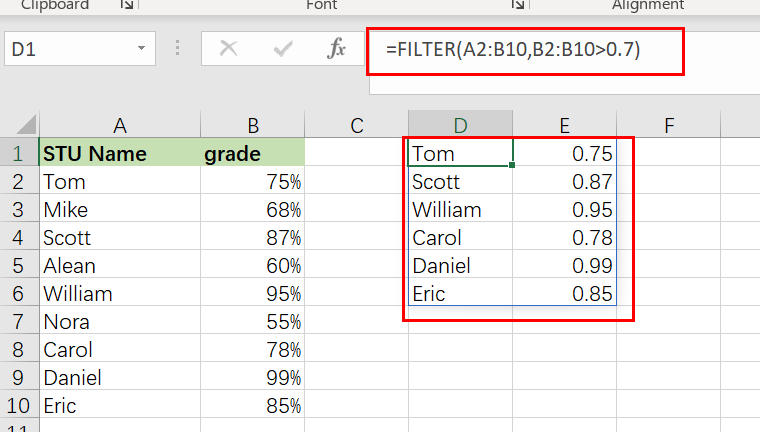### Example 2: How to filter in Excel by a cell value

In this excel filter function example, we want to do the same thing as stated before, but rather than inputting the condition straight into the formula, we’re going to use a cell reference.

When you filter in Excel by a cell value, your sheet is configured in such a way that you may alter the value in the cell at any moment, which updates the value to which the filter criterion is tied.

In this example, rather than explicitly entering the value “0.8” into the formula, the filter criterion is set to cell G1, which contains the “`0.8`” value.

The assignment: Display a list of students and their grades, but only those with a score of less than `80%`.

The reasoning: Filter the range A2:B10 to the extent that B2:B10 is smaller than the value supplied in column G1 (0.8).

You can use the following FILTER formula, type:

`=FILTER(A2:B10, B2:B10 <G1)`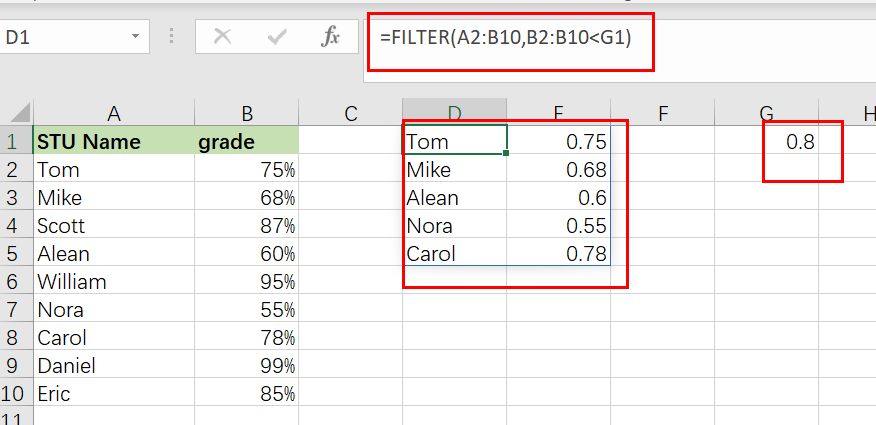### Example 3: Using Excel’s text filter

In this example, we’ll utilize a text string as the filter formula’s criterion. This is fairly similar to using a number, except that the text to filter must be enclosed in quote marks.

We are filtering a list of customers and their payment status in this instance, and we want to present just customers with a payment status of “`Payed`“.

The objective is to provide a list of clients that have paid on their payments.

The reasoning: Filter the range A2:B6 by substituting the string “` Payed` ” for B2:B6.

The following formula: In this example, the formula below is typed in the cell (E1).

`=FILTER(A3:B12, B2:B6=" Payed ")`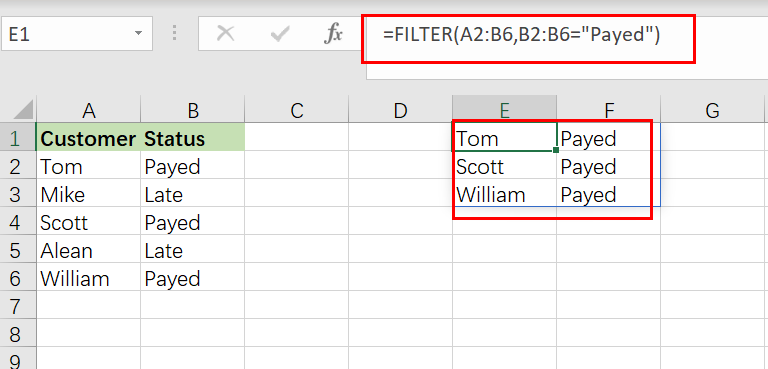### Example 4: Using NOT EQUAL TO as a FILTER condition in Excel

Now that you have a working knowledge of how to use the filter function in Excel, here is another example of filtering by a string of text, but this time we will use the “not equal” operator (<>) to demonstrate how to filter a range and return data that is NOT equal to the criteria you set.

Additionally, we will utilize a bigger data set in this example to show a more comprehensive usage of the FILTER function in the real world.

You may be surprised at how often a circumstance arises in which you need to filter data that is “`not equal to`” a certain number or piece of text.

In this example, we’ll use a report/spreadsheet to display data from sales calls that occur at your organization, and we’ll filter the data to exclude a certain sales person (Scott) from the result.

The assignment: Display sales call statistics for all sales representatives except ” Scott “.

The reasoning: Filter the range `A2:C10` for values `A2:A10` that DO NOT match the string “Scott “.

The following formula: In this example, the formula below is typed in the cell (E1).

`=FILTER(A2:C10, A2:A10 <>"Scott")`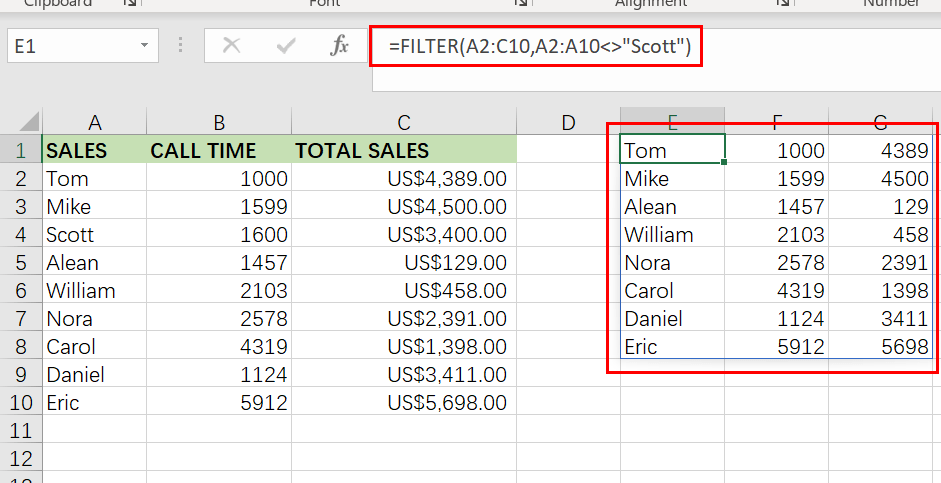Take note that the filtered data on the right side of the figure above does not include any of `Scott `‘s rows/calls.

### Example 5: How to use the date filter in Excel

Filtering in Excel by a date may be accomplished in a few different methods, which I will demonstrate below. If you attempt to put a date into the FILTER function in the same way that you would typically type into a cell, the formula will fail to operate properly.

Therefore, you may either enter the date you want to filter into a cell and then reference that cell in your formula… Alternatively,  you may use the DATE function.

When filtering by date, the same operators (>, =, etc…) are available as they are in other FILTER function applications. Each individual day/date in Excel is merely a number that has been formatted differently. In Excel, for example, the date “`01/30/2022`” is just the serial number “`44591`” formatted as a date. Each time you add a day to the calendar, this number increases by one… For example, “44591” “44592” “44593”

Thus, if one date is farther in the future, it might be regarded “`greater than`” another. In contrast, if one date is farther in the past, it might be considered to be “`less than`” another.

In this example, we’ll use a cell reference to filter on a date. This is identical to the example discussed in Example 2, except that we are dealing with dates instead of percentages.

Consider the following scenario: we want to filter a list of students, their exam results, and the dates on which the tests were administered… and we wish to display only tests conducted before to June (05/01/2022).

`=FILTER(A2:C10, C2:C10 <G1)`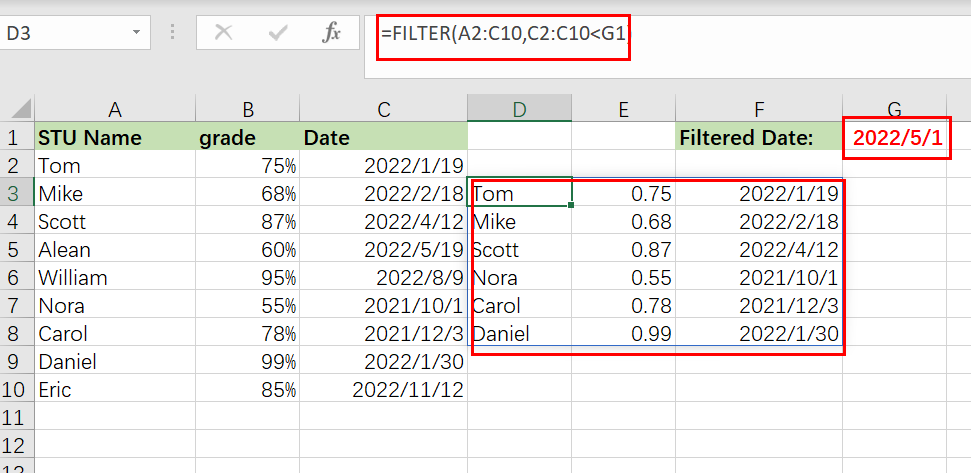### Example 6: Filtering by date in Excel

In this example of date filtering in Excel, we’ll use the same data as in the previous one and attempt to obtain the same results… however, instead of referencing a cell, we’ll utilize the DATE function, which allows you to put the date straight into the FILTER function.

When using the DATE function to provide a date, you must first input the year, followed by the month and finally the day… each denoted with a comma (shown below).

The assignment: Display only exams given before to May

The reasoning: Filter the range `A2:C10 `so that `C2:C10 `is less than or equal to the date (05/01/2022).

The following formula: In this example, the formula below is typed in the cell (D3).

`=FILTER(A2:C10,C2:C10<DATE(2022,5,1))`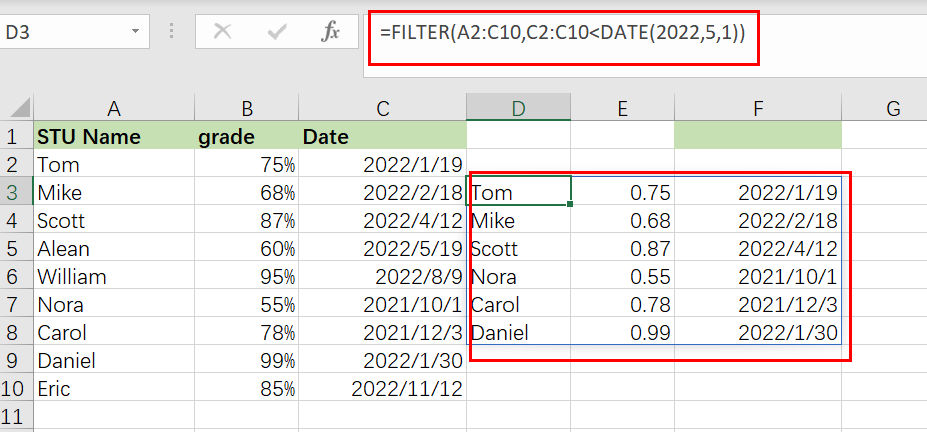### Example 7: Filtering based on two Conditions

When utilizing the Excel FILTER function, you may want to produce data that fits many criteria. I’ll demonstrate two methods for filtering by several criteria in Excel, depending on the scenario and the desired behavior of the calculation.

The conventional method of adding another condition to your filter function (as shown by the Excel formula syntax) allows you to provide a second condition, where both the first AND second conditions must be fulfilled in order for the filter output to be returned.

However, I will demonstrate how to make a little tweak to the function so that you may choose to return/display in the filter function’s output/destination a second condition where EITHER condition might be satisfied. (To utilize AND logic, separate the conditions with an asterisk, or use a plus symbol to separate the criteria.)

In this example, we’re going to filter a collection of data and show those rows that satisfy BOTH the first and second conditions.

To utilize a second condition in this manner (using AND logic), just insert it after the first condition in the formula, separated by an `asterisk (*)`. Each condition must be included in a separate pair of parentheses.

When a filter formula is used with several conditions, the columns referenced in each condition must be distinct.

In this case, we’d want to filter a list of clients based on their payment status and region… and to display those customers who are both current members AND paid on their payment status.

The objective is to provide a list of customers who are paid on payments, but only those who are in East region.

The reasoning: Filter the range A2:C6 such that B2:B6 equals the text “`Paid`,” AND C2:C6 equals the text “`East`“.

The following formula: In this example, the formula below is typed in the blue cell (E1).

`=FILTER(A2:C6,( B2:B6="Paid")*( C2:C6 ="East"))`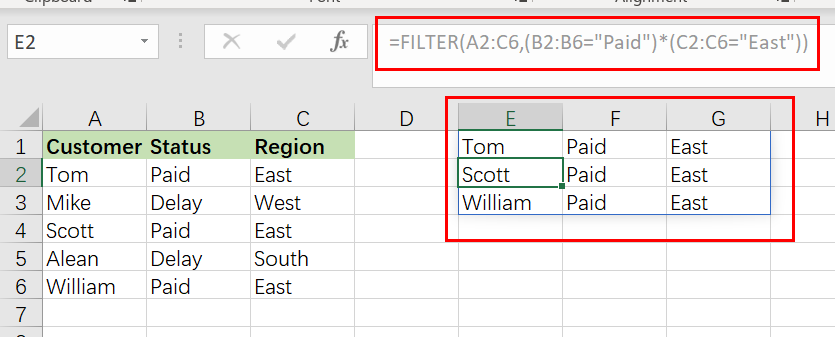### Example 8: Filtering Based on Two Conditions using OR Logic

In this example, we’re going to filter a collection of data and show those rows that satisfy either the first OR the second criterion.

To utilize a second condition in this manner (using OR logic), just insert it after the first condition in the formula, separated by a `plus sign`. Each condition must be included in a separate pair of parentheses (shown below).

When used in this manner, the FILTER formula allows you to choose criteria from the same or separate columns.

In this case, we’re filtering the same customer data as in the previous example, but this time we’re displaying a list of customers who either are in `East` region OR have paid on a payment. This will generate a list of clients to whom a payment notification have paid… whether they are current members or east region who have paid on their last payment.

The objective is to provide a list of customers who are in `East` region, as well as customers who have paid on payments regardless of whether they are in East region.

The following formula: In this example, the formula below is typed in the cell (E1).

`=FILTER(A2:C6, (B2:B6="Paid ")+(C2:C6=" East ")`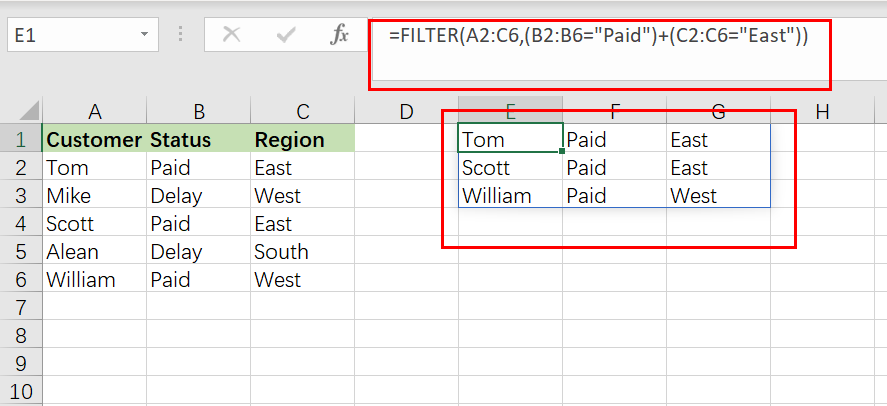### Example 9: Filter Data in Excel from Another Sheet

You may often encounter instances in Excel when you need to filter data from another sheet, where your raw unfiltered data is on one tab and your filter formula is on another sheet.

This may be accomplished by simply referring to a certain sheet’s name when providing the filter’s ranges. Thus, while you would typically give a range such as “A1:B4,” when referring another sheet when filtering, you indicate the sheet name by preceding the range with the sheet name and an exclamation mark, as in “`SheetName!A1:B4`“.

However, if the sheet name contains a space, an apostrophe must be used before and after the sheet name, as in `"Sheet Name!" A1:B4`.

The following is an example of how to filter data in Excel from a separate sheet, where the filter formula is located on a different sheet than the source range.

Consider the following scenario: On one sheet, you have a list of customers and their payment status, and you wish to present a filtered list of  paid customer on another sheet.

The job is to filter the list of customers on the Sheet3 and to display a separate list of customer names with a pay status on another worksheet.

The reasoning: Filter the range using the `Sheet3` command! A2:C6, where ‘ Sheet3′ is the range! B2:B6 corresponds to the phrase “Paid“.

The following formula: In this example, the formula below is typed in the cell (A3).

`=FILTER(Sheet3! A2:C6, Sheet3!B2:B6="Paid")`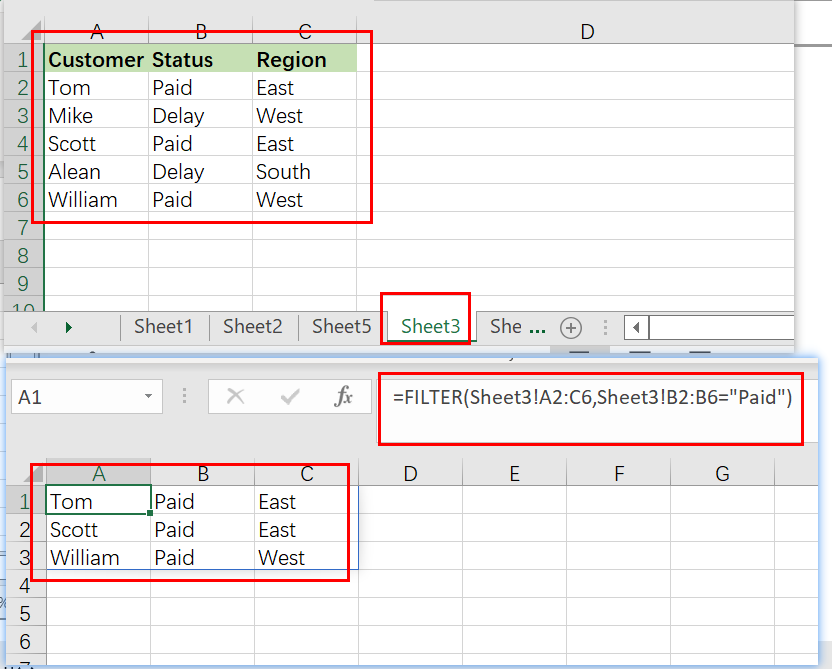### Example 10: Providing Maximum Number of Rows of Filtered Data

If your FILTER formula provides a large number of rows but your worksheet is restricted in space and you are unable to erase the data below, you may limit the amount of rows returned by the FILTER function.

Let us demonstrate how it works using a simple formula that filter data that grade is less than 0.7 from filter value in Cell F1:

`=FILTER(A2:C10, B2:B10<F1)`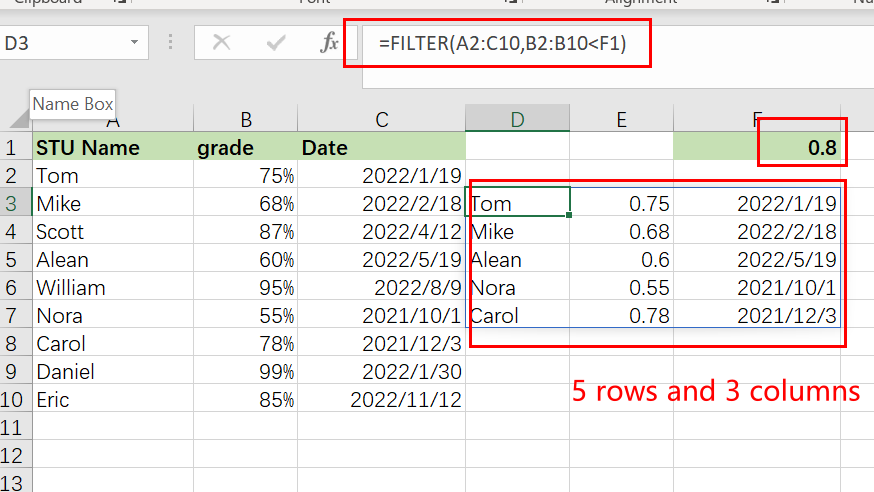The preceding formula produces all records that it discovers, in this instance five rows. However, imagine you only have room for two. To output just the first two rows discovered, follow these steps:

Step1: Incorporate the FILTER formula into the INDEX function’s array parameter

Step2: Use a vertical array constant such as 1;2 as the row num input to INDEX. It specifies the number of rows to return (2 in our case).

Step3: Use a horizontal array constant such as 1,2 for the column num parameter. It defines the columns that should be returned (the first 2 columns in this example).

Step4: To account for any mistakes caused by the absence of data meeting your criteria, you may wrap your calculation in the IFERROR function.

The entire excel filter formula is as follows:

`=IFERROR(INDEX(FILTER(A2:C10, B2:B10<F1), {1;2},{1,2}), "No Found")`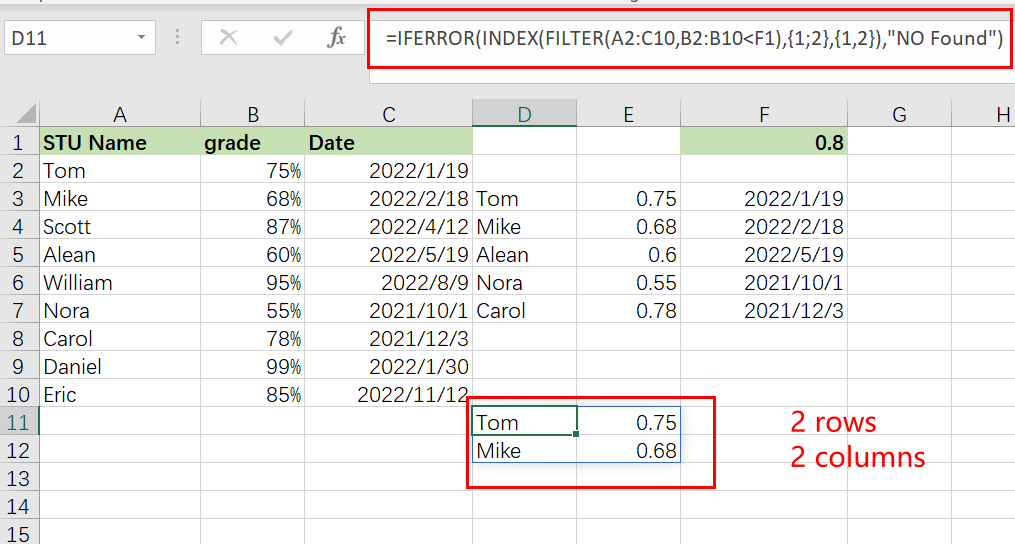## Conclusion

This section discusses the FILTER function and its many uses. In general, when it comes to time management, we need this feature for a variety of reasons. I demonstrated various techniques with accompanying examples, however there might be countless further iterations based on a variety of circumstances. If you know of another way to use this function, please share it with us.

### Related Functions

• Excel IFERROR function
The Excel IFERROR function returns an alternate value you specify if a formula results in an error, or returns the result of the formula.The syntax of the IFERROR function is as below:= IFERROR (value, value_if_error)….
• Excel INDEX function
The Excel INDEX function returns a value from a table based on the index (row number and column number)The INDEX function is a build-in function in Microsoft Excel and it is categorized as a Lookup and Reference Function.The syntax of the INDEX function is as below:= INDEX (array, row_num,[column_num])…

Related Posts

Break ties with helper COUNTIF and column

Suppose you got a task to adjust the values that contain the ties; what would be your first attempt to break the ties of the given value? If you are wondering about doing this task manually, let me add that ...

Find the Closest Data to the Data Provided in Excel

In our daily work, we may encounter such an issue that to find the closest value to a certain value. In fact, Excel internal functions can help us solve this problem. In today’s article, we will show you how to ...

Creating a Table with Automatic Row Numbering

When creating an Excel Table, you may use a calculation relying on the ROW function to insert row numbers automatically. The formula in A2, which has been copied down, is as follows: =ROW()-ROW(Table1[#Headers]) Note: the default table name is Table1. ...

Get Address of First Cell in Range

We will learn how to get address of first cell in range in this post. At Instance, we must determine the address of first cell in range to use as the data table's value. For the DATA table, we have ...

Convert State Names To Abbreviations

Assume that you got a task to convert the full state's name into the abbreviations in MS Excel, and for doing this task, you might choose to do it manually, which is an acceptable way only if you don't have ...

Sidebar